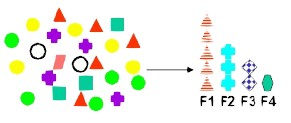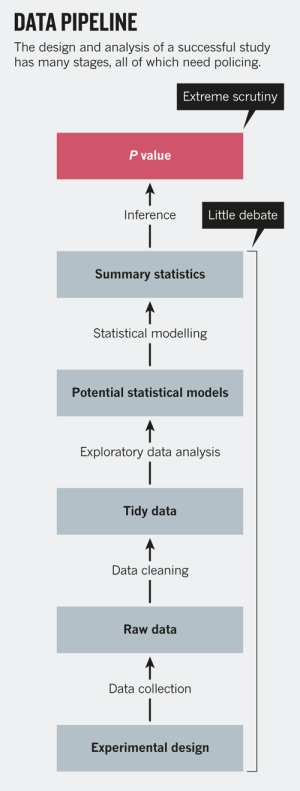# Data Mining - Principal Component (Analysis|Regression) (PCA|PCR)

Principal Component Analysis (PCA) is a feature extraction method that use orthogonal linear projections to capture the underlying variance of the data.

By far, the most famous dimension reduction approach is principal component regression. (PCR).

PCA can be viewed as a special scoring method under the SVD algorithm. It produces projections that are scaled with the data variance. Projections of this type are sometimes preferable in feature extraction to the standard non-scaled SVD projections.

The PCR idea is to summarize the features by the principle components, which are the combinations with the highest variance.

## Principal component

The principal components of a collection of points is the direction of a line that best fits the data while being orthogonal to the first vectors. The fit process minimizes the average squared distance from the points to the best line.

PCA can be thought of as fitting a p-dimensional ellipsoid to the data, where each axis of the ellipsoid represents a principal component. If some axis of the ellipsoid is small, then the variance along that axis is also small.

In dimensionality reduction, the goal is to retain as much of the variance in the dataset as possible. The more along the axis, the better,

## Assumption

When PCR compute the principle components is not looking at the response but only at the predictors (by looking for a linear combination of the predictors that has the highest variance). It makes the assumption that the linear combination of the predictors that has the highest variance is associated with the response.

When choosing the principal component, we assume that the regression plane varies along the line and doesn't vary in the other orthogonal direction. By choosing one component and not the other, we're ignoring the second direction.

PCR looks in the direction of variation of the predictors to find the places where the responses is most likely to vary.

With principal components regression, the new transformed variables (the principal components) are calculated in a totally unsupervised way:

• the response Y is not used to help determine the principal component directions).
• the response does not supervise the identification of the principal components.
• PCR just looks at the x variables

We're just going to cross our fingers that the directions on which the x variables really vary a lot are the same directions in which the variables are correlated with the response y.

There is no guarantee that the directions that best explain the predictors will also be the best directions to use for predicting the response.

To perform an PCA analyse on a supervised way, we can instead perform partial least squares.

## Steps

### Getting the principal components

Getting the principal components of the data matrix x.

Procedure:

• The first principle component is just the normalized linear combination of the variables that has the highest variance.
• The second principal component has largest variance, subject to being uncorrelated with the first.
• And so on.

The principal components produces a linear combinations or dimensions of the data that are really high in variance and that are uncorrelated.

#### Example

• The direction in which the data varies the most actually falls along the green line. This is the direction with the most variation in the data, this is why it's the first principal component (direction). The sum of square distances is the smallest possible.
• What's the direction along which the data varies the most out of all directions that are uncorrelated with the first direction? That's this blue dashed line. That's the second principle component in this data.

#### Principal Component Plot

A plot of the principle components against the variable helps to understand them better.

As the first principle component is highly correlated with all variables, it means that it summarizes the data very well. Then instead of using the variables X or Y to make prediction, we can use just the first principle component.

When two variables are really correlated with each other, one new variable (ie the first principle component) can really summarize both of those two variables very well.

### Fitting the models with the principal components

1. perform least squares regression using those principal components as predictors.

### Choosing M, the number of principal component (or directions)

When more and more components are used in the regression model, the bias will decrease (because we fit more and more complex model) but the variance will then increase.

where:

• MSE has a U shape and is smallest for a model with around 18 principal components.

Cross validation was chosen in order to choose the number of principal component directions.

where:

• With 11 components, we got the full least squares model because when m equals p this is regular least squares on the original data.
• The cross validated mean squared error is an estimate of the test error.

In the below graphic, the mean square error is the smallest for 10 or 11 components which corresponds to least squares. Then Principal components regression doesn't give any gains over just plain least squares. Doing least squares on the original data is the best option.

## Documentation

Recommended PagesData Mining - (Feature|Attribute) Extraction Function

Feature extraction is the second class of methods for dimension reduction. dimension reduction It creates new attributes (features) using linear combinations of the (original|existing) attributes. ...Data Mining - (Life cycle|Project|Data Pipeline)

Data mining is an experimental science. Data mining reveals correlation, not causation. With good data, you will make good algorithm. The most preferable solution is then to work on good features....Data Mining - Partial least squares (PLS)

Partial least squares (PLS) is is a dimension reduction method and uses the same method than principle components regression but it selects the new predictors (principal component) in a supervised way....Machine Learning - Unsupervised Learning ( Mining )

Unsupervised learning is the second type of function that an algorithm can perform. The algorithm is said to be unsupervised when no response is used in the algorithm. Unsupervised Learning has the goal...Notion - (Best approximation|Closest|Closeness)

This notion of “best approximates” comes up again and again: in least-squares, a fundamental data analysis technique, image compression, in principal component analysis, another data analysis...R - Principal Component Analysis

in R. Package stats where: scale=TRUE will standardize the variables in order to take into account the different unity. The data The variables associated (names) Unclass will show...Statistics

is a scientific discipline devoted to the study of data. is the art of extracting information from data. From Data to Information to Knowledge. No learning. lies lies, damned lies, and statistics....Statistics - Factor Analysis

Factor analysis is a feature extraction statistical method used to describe variability among observed, correlated variables in terms of a potentially lower number of unobserved variables called factors....Statistics - Singular Value Decomposition (SVD)

Singular Value Decomposition (SVD) is a feature extraction methods that use orthogonal linear projections to capture the underlying variance of the data. The SVD projections are not scaled with the data...